# Close Packing

(redirected from Close-packing)
Also found in: Dictionary, Thesaurus, Wikipedia.
The following article is from The Great Soviet Encyclopedia (1979). It might be outdated or ideologically biased.

## Close Packing

in crystallography, the arrangement of atoms in the crystal lattice characterized by the largest number of atoms per unit volume of the crystal. Close packing is distinctly expressed in a large number of crystal structures; it is characteristic of most metals and of crystallized inert gases. The structures of many inorganic (ionic) crystals are close packings of spherical anions (with large ionic radii) in whose cavities small cations are distributed.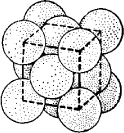Figure 1. Cubic close packing. Unit cell.

For more than 300 years—since the studies of J. Kepler—the packing arrangement for spheres recognized as the densest possible arrangement was that shown in Figure 1. Here, a layer of spheres in a square arrangement is superimposed on another such layer, with the upper spheres fitted to the cavities in the lower layer. The percentage of filled space is 74.05 percent (Figure 2).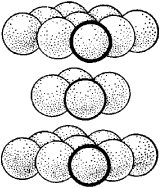Figure 2. Layers with square symmetry that generate cubic close packing

It is evident that the spheres of the third layer will lie exactly above the spheres of the first. This packing is usually called a face-centered cubic structure. It was considered unique until the English crystallographer W. Barlow demonstrated in 1900 that if a cube is positioned at an angle, it can be disassembled into even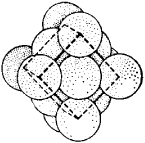Figure 3. Layers with hexagonal symmetry that generate close packing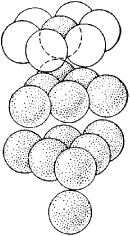Figure 4. Hexagonal close packing

denser layers (Figure 3), in which the number of cavities between spheres would be twice as great as the number of spheres themselves (Figure 4). If the arrangement of close-packed layers is varied (Figure 5), an infinite number of close packings can be obtained; in each arrangement the percentage of filled space isFigure 5. Close-packed layer with the number of cavities twice the number of spheres

74.05 percent. If the superimposition sequence is limited to some period, the following are obtained: a two-layer close packing (Figure 6,a; the third layer duplicates the first), a three-layer close packing (Figure 6,b; the fourth layer duplicates the first), a four-layer close packing (Figure 6,c), and so forth. The three-layer packing is the original cubic structure, and all others are hexagonal.

The noble metals Ag, Au, and Pt and the metals Cu, Al, Pb, and γ-Fe are characterized by a three-layer, cubic close packing.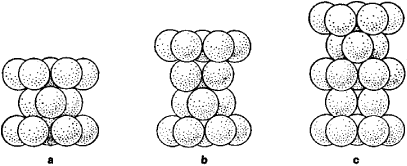Figure 6. Close packing of spheres: (a) two-layer, (b) three-layer, (c) four-layer

Be, Mg, Zn, and Ti have two-layer packing, and such rare-earth metals as La and Ce have four-layer packing. Polymorphism—not only of pure metals but also of compounds with the simplest formula AX—very often reduces to the replacement of close packing by 6-, 8-, and 15-layer packing all the way to several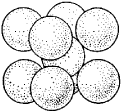Figure 7. Body-centered packing

tens of layers, as in the case of carborundum (SiC). The crystal lattices of some compounds are characterized by a less dense body-centered packing (Figure 7) with 68 percent of the volume filled (α-iron and the alkali metals).

Since two- and three-layer packing with the standard arrangement of anionic spheres is the most common, the structure of a chemical compound is often determined by the distribution of other structural elements, chiefly cations, throughout the cavities of the close-packed structures. There are two types of cavities: those found among six spheres (octahedral) and those found among four spheres (tetrahedral); there are twice as many of the latter as of the former (as shown in Figure 8, with the anions at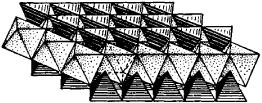Figure 8. A layer of close-packed octahedrons and tetrahedrons In a ratio of 1:2

the vertices of close-packed polyhedrons). The description of the structures is usually limited to the identification of filled polyhedrons in these layers. The polyhedrons are colored or shaded differently according to the types of atoms filling them (Figure 9).Figure 9. Three-way shading of filled tetrahedrons in the close-packed structure of stannite (Cu2FeSnS4)

### REFERENCE

Belov, N. V. Struktura ionnykh kristallov i metallicheskikh faz. Moscow, 1947.

N. V. BELOV

The Great Soviet Encyclopedia, 3rd Edition (1970-1979). © 2010 The Gale Group, Inc. All rights reserved.
Site: Follow: Share:
Open / Close